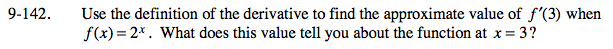### Home > PC > Chapter 9 > Lesson 9.3.4 > Problem9-142

9-142.

Use the definition of the derivative to find the approximate value of f ′(3) when f(x) = 2x. What does this value tell you about the function at x = 3? Homework Help ✎Testing small values of h yields f '(3) ≈ 5.545. The slope of the function is 5.545 at 3 or the slope of tangent line at x = 3 is 5.545.
This tells you the function is increasing at that point.